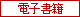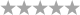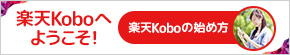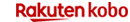トップ > 電子書籍 > 洋書 > Science & Nature > Statistics

# Stochastic Analysis　Itô and Malliavin Calculus in Tandem　（Cambridge University Press）　[電子書籍版] Hiroyuki Matsumoto

• ユーザ評価0件）
•  | レビューを書く

5,356円（税込）## 商品説明

Thanks to the driving forces of the Itô calculus and the Malliavin calculus, stochastic analysis has expanded into numerous fields including partial differential equations, physics, and mathematical finance. This book is a compact, graduate-level text that develops the two calculi in tandem, laying out a balanced toolbox for researchers and students in mathematics and mathematical finance. The book explores foundations and applications of the two calculi, including stochastic integrals and differential equations, and the distribution theory on Wiener space developed by the Japanese school of probability. Uniquely, the book then delves into the possibilities that arise by using the two flavors of calculus together. Taking a distinctive, path-space-oriented approach, this book crystallizes modern day stochastic analysis into a single volume.

まだレビューがありません。

## 楽天ブックスランキング情報

•週間ランキング

ランキング情報がありません。

•日別ランキング

ランキング情報がありません。

## ご購入はこちらから電子書籍版

5,356円（税込）

2018/3/25(日) 23:59まで

エントリー＆楽天カード利用で
ポイント5倍

2018/3/30(金) 23:59まで
25,000円以上購入で使える！
40%OFF
5と0のつく日は楽天Koboがお得に！

## 最近チェックした商品

※表示を削除したい場合は閲覧履歴のページから削除お願いいたしますこのページの先頭へ